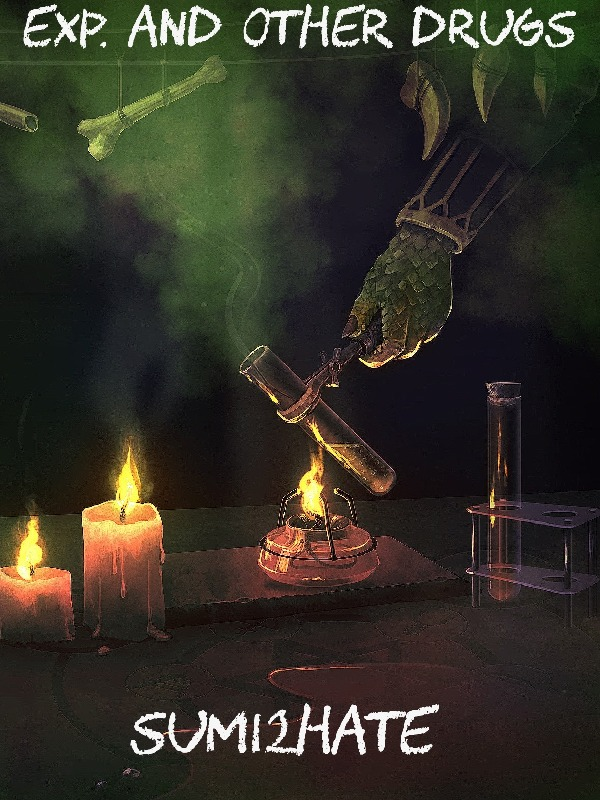# Reviews of Exp and other drugs### Exp and other drugs

Sum12hate

• Overall Rate
• Writing Quality
• Updating Stability
• Story Development
• Character Design
• world background

## Reviews11HalonFox1Lv11HalonFox1

It may be a little early to review for most however I find the level an style of writing I've seen to be enough for me to decide that this is going to be fun The main character is a druid humanoid that was transmigrated by Bahamut and birthed with the blood of Tiamat the biggest badest dragons around but even with all that OP edge the main character manages to stay likable and the cast is being fleshed out amazingly as we go along. I hope anyone who reads this will decide to join me on this wild ride our kind author has decided to write for us Hopefully, see you in the comments and Bye for Now!!!!

Become a KOL for my discussion channel!

Engage with others on the app, and become a moderator for my discussion channel. Let this be a place for sharing with other fellow bookworms!Rex_SalamanderLv5Rex_Salamander

Exp Exp Exp Exp Exp Exp Exp Exp Exp Exp Exp Exp Exp Exp Exp Exp Exp Exp Exp Exp Exp Exp Exp Exp Exp Exp Exp Exp Exp Exp Exp Exp Exp Exp Exp Exp Exp Exp Exp Exp Exp Exp Exp Exp Exp Exp Exp Exp Exp Exp Exp Exp Exp ExpRex_SalamanderLv5Rex_Salamander

Exp Exp Exp Exp Exp Exp Exp Exp Exp Exp Exp Exp Exp Exp Exp Exp Exp Exp Exp Exp Exp Exp Exp Exp Exp Exp Exp Exp Exp Exp Exp Exp Exp Exp Exp Exp Exp Exp Exp Exp Exp Exp Exp Exp Exp Exp Exp Exp Exp Exp Exp Exp Exp Exp Exp Exp Exp Exp Exp Exp Exp Exp Exp Exp Exp Exp Exp Exp Exp Exp Exp ExpRex_SalamanderLv5Rex_Salamander

Exp Exp Exp Exp Exp Exp Exp Exp Exp Exp Exp Exp Exp Exp Exp Exp Exp Exp Exp Exp Exp Exp Exp Exp Exp Exp Exp Exp Exp Exp Exp Exp Exp Exp Exp Exp Exp Exp Exp Exp Exp Exp Exp Exp Exp Exp Exp Exp Exp Exp Exp Exp Exp Exp Exp Exp Exp Exp Exp Exp Exp Exp Exp Exp Exp Exp Exp Exp Exp Exp Exp ExpRex_SalamanderLv5Rex_Salamander

Exp Exp Exp Exp Exp Exp Exp Exp Exp Exp[img=exp][img=exp][img=exp][img=exp][img=exp][img=exp][img=exp][img=exp][img=exp][img=exp][img=exp][img=exp][img=exp][img=exp][img=exp][img=exp][img=exp]Rex_SalamanderLv5Rex_Salamander

EXP EXP EXP EXP EXP EXP EXP EXP EXP EXP EXP EXP EXP EXP EXP EXP EXP EXP EXP EXP EXP EXP EXP EXP EXP EXP EXP EXP EXP EXP EXP EXP EXP EXP EXP EXP EXP EXP EXP EXPThesoultaker88Lv5Thesoultaker88

exp exp exp exp exp exp exp exp exp exp exp exp exp exp exp exp exp exp exp exp exp exp exp exp exp exp exp exp exp exp exp exp exp exp exp exp exp exp exp exp exp exp exp exp exp exp exp exp exp exp exp exp exp exp exp exp exp exp exp exp exp exp exp exp exp exp exp exp exp exp exp expshashank_bhattLv13shashank_bhatt

Exp Exp Exp Exp Exp Exp Exp Exp Exp Exp Exp Exp Exp Exp Exp Exp Exp Exp Exp Exp Exp Exp Exp Exp Exp Exp Exp Exp Exp Exp Exp Exp Exp Exp Exp Exp ExpKenlinvert1Lv1Kenlinvert1

Hi there! Are you looking for inspiration? You might want to check out our Prompts Writing contest! Apocalypse, Isekai, LitRPG... Follow the three provided prompts and take the chance to win up to \$2,000! Please Google 70daysthemedwritingchallenge to find out more! This contest is free entry and open to any writer at any country. If you had any query, please feel free to contact ringdom_promptswriting@hotmail.com Good luck for writing!KenringdomstorysLv1Kenringdomstorys

Hey there! Here are some web novel recommendations for whom share the same taste with me. If you like this story, my recommendations are worth a try! https://docs.google.com/document/d/19R5KkqHOOHSMDkF4fHscVmxWcYGh0OntYsv8NglzsQo/edit?usp=sharingKen_RingdomstoryLv1Ken_Ringdomstory

Hey there! Good day for writing! If you wanted to see whether you can get paid by distributing the current work or getting financial support by writing new work, you might want to contact kenreview@outlook.com. A brief introduction, some sample chapters or links will be appreciated when reaching out.